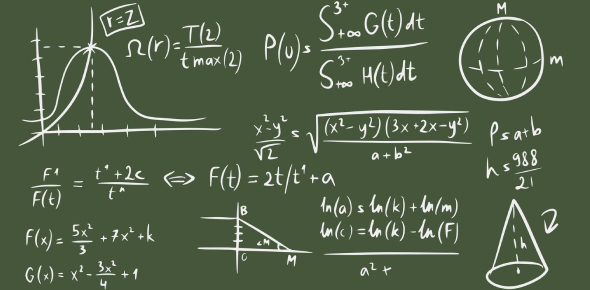# Composition Of Functions Questions And Answers

10 Questions | Attempts: 545
ShareSettingsDo you know how to find the composition of functions? Can you solve these function composition questions and answers in this quiz? Go ahead then and check how good you are at solving mathematics problems. In mathematics, the composition of functions is an operation that is written inside another function. It takes two functions f and generates a new function; we can say h in such a way that h(x) = g(f(x)). Here, you have to solve function composition problems. Let's see if you can do that or not.

• 1.
Find the value of f(6) in f(x)=x²+3x-2
• 2.
An electrician charges a base fee of \$70 plus \$50 for each hour of work. Write a function that shows the amount the electrician charges for every he works.
• 3.
Given functions f(x) = 3x+½   and g(x)= 7+2x. Find f×g.
• A.

F(x)=6x²+21x+2½

• B.

F(x)=6x²+22x+3½

• C.

F(x)=5x²+20x+2½

• D.

None of these

• 4.
Given functions f(x) = 3x+½   and g(x)= 7+2x. Find f-g.
• A.

F(x)=x-6½

• B.

F(x)=x-5½

• C.

F(x)=2x-6½

• D.

F(x)=x+6½

• 5.
Given functions f(x) = 3x+½   and g(x)= 7+2x. Find f/g.
• A.

F/g= (3x+1) /(7+2x)

• B.

F/g= (3x+½) /(7+2x)

• C.

F/g= (3x+½) /(6+2x)

• D.

F/g= (3x-1) /(7+2x)

• 6.
Given functions f(x) = 3x+½   and g(x)= 7+2x. Find f ∘ g.
• A.

F ∘ g= 7x+21 ½

• B.

F ∘ g= 6x+21

• C.

F ∘ g= 6x+21 ½

• D.

None of these

• 7.
Given functions f(x) = 3x+½   and g(x)= 7+2x. Find g ∘ f.
• A.

G ∘ f=6x+8

• B.

G ∘ f=6x+9

• C.

G ∘ f=x+8

• D.

G ∘ f=x-8

• 8.
Given functions f(x) = 3x+½   and g(x)= 7+2x. Find f+g.
• A.

F+g=5x+7 ½

• B.

F+g=x+7 ½

• C.

F+g=5x+7

• D.

F+g=5x-7 ½

• 9.
For f(x) = 2x + 3 and g(x) = -x 2 + 1, find (f o g)(x)
• A.

- 2x2+5

• B.

2x2+5

• C.

- 2x2+7

• D.

- 2x2-5

• 10.
find (g∘f)(x) for x = 2 if f(x) = 2x +1 and g(x) = -x2.
• A.

20

• B.

21

• C.

25

• D.

26

## Related TopicsBack to top
×

Wait!
Here's an interesting quiz for you.